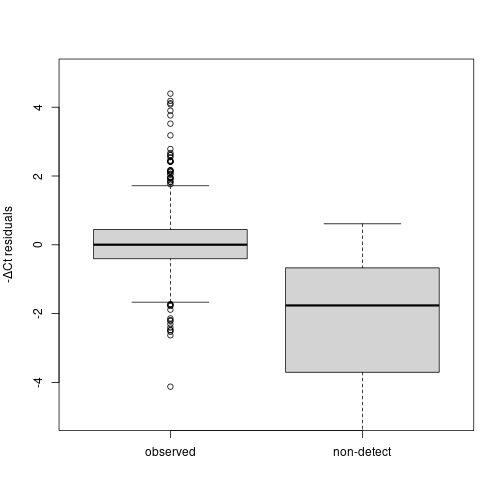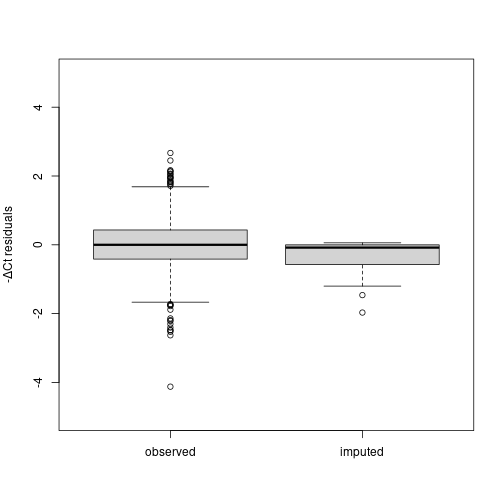# 1 Background on non-detects in qPCR data

Quantitative real-time PCR (qPCR) measures gene expression for a subset of genes through repeated cycles of sequence-specific DNA amplification and expression measurements. During the exponential amplification phase, each cycle results in an approximate doubling of the quanitity of each target transcript. The threshold cycle (Ct) – the cycle at which the target gene's expression first exceeds a predetermined threshold – is used to quantify the expression of each target gene. These Ct values typically represent the raw data from a qPCR experiment.

One challenge of qPCR data is the presence of non-detects those reactions failing to attain the expression threshold. While most current software replaces these non-detects with the maximum possible Ct value (typically 40), recent work has shown that this introduces large biases in estimation of both absolute and differential expression. Here, we treat the non-detects as missing data, model the missing data mechanism, and use this model to impute Ct values for the non-detects.

# 2 A statistical model for qPCR non-detects

We propose the following generative model for qPCR data in which $$Y_{ij}$$ is the measured expression value for gene $$i$$ in sample $$j$$, some of which are missing (non-detects), $$X_{ij}$$ represents the fully observed expression values, and $$Z_{ij}$$ indicates whether a value is detected: $X_{ij} = f(\theta_{ij}, \eta) + \varepsilon_{ij}$ $Y_{ij} = \left\{ \begin{array} {rr} X_{ij} & \textrm{if Z_{ij}=1}\ \textrm{non-detect} & \textrm{if Z_{ij}=0} \end{array} \right.$

In this model, we assume that the fully observed expression values, $$X_{ij}$$ are a function of the true gene expression, $$\theta_{ij}$$, non-biological factors, $$\eta$$, and random measurement error, $$\varepsilon_{ij}$$. The probability of an expressed value being detected is assumed to be a function of the expression value itself, $$g(X_{ij})$$, for values below the detection limit, $$S$$. The following logistic regression model is a natural choice for such a relationship:

$Pr(Z_{ij}=1) = \left\{ \begin{array} {rr} g(X_{ij}) & \textrm{if X_{ij} < 40} \\ 0 & \textrm{otherwise} \end{array} \right.$

Here, $$g(X_{ij})$$ can be estimated via the following logistic regression: $logit(Pr(Z_{ij}=1)) = \beta_0 + \beta_1 X_{ij}$

# 3 Example

## Data from Sampson et al. Oncogene 2013

Two cell types – young adult mouse colon (YAMC) cells and mutant-p53/activated-Ras transformed YAMC cells – in combination with three treatments – untreated, sodium butyrate, or valproic acid. Four replicates were performed for each cell-type/treatment combination [ @sampson2012gene].

library(HTqPCR)

## Loading required package: Biobase

## Loading required package: BiocGenerics

##
## Attaching package: 'BiocGenerics'

## The following objects are masked from 'package:stats':
##
##     IQR, mad, sd, var, xtabs

## The following objects are masked from 'package:base':
##
##     Filter, Find, Map, Position, Reduce, anyDuplicated, append,
##     as.data.frame, basename, cbind, colnames, dirname, do.call,
##     duplicated, eval, evalq, get, grep, grepl, intersect, is.unsorted,
##     lapply, mapply, match, mget, order, paste, pmax, pmax.int, pmin,
##     pmin.int, rank, rbind, rownames, sapply, setdiff, sort, table,
##     tapply, union, unique, unsplit, which.max, which.min

## Welcome to Bioconductor
##
##     Vignettes contain introductory material; view with
##     'browseVignettes()'. To cite Bioconductor, see
##     'citation("Biobase")', and for packages 'citation("pkgname")'.

## Loading required package: RColorBrewer

## Loading required package: limma

##
## Attaching package: 'limma'

## The following object is masked from 'package:BiocGenerics':
##
##     plotMA

library(mvtnorm)
library(nondetects)
data(oncogene2013)


## Examine residuals when non-detects are replaced by 40

Normalize to Becn1:

normCt <- normalizeCtData(oncogene2013, norm = "deltaCt", deltaCt.genes = "Becn1")

## Calculating deltaCt values
##  Using control gene(s): Becn1
##  Card 1: Mean=26.17  Stdev=NA
##  Card 2: Mean=25.5   Stdev=NA
##  Card 3: Mean=25.85  Stdev=NA
##  Card 4: Mean=26.22  Stdev=NA
##  Card 5: Mean=26.59  Stdev=NA
##  Card 6: Mean=25.35  Stdev=NA
##  Card 7: Mean=25.73  Stdev=NA
##  Card 8: Mean=26.36  Stdev=NA
##  Card 9: Mean=26.13  Stdev=NA
##  Card 10:    Mean=25.38  Stdev=NA
##  Card 11:    Mean=25.61  Stdev=NA
##  Card 12:    Mean=26.52  Stdev=NA
##  Card 13:    Mean=26.12  Stdev=NA
##  Card 14:    Mean=26.56  Stdev=NA
##  Card 15:    Mean=26.02  Stdev=NA
##  Card 16:    Mean=25.5   Stdev=NA
##  Card 17:    Mean=25.76  Stdev=NA
##  Card 18:    Mean=26.03  Stdev=NA
##  Card 19:    Mean=26.67  Stdev=NA
##  Card 20:    Mean=26.69  Stdev=NA
##  Card 21:    Mean=26.11  Stdev=NA
##  Card 22:    Mean=25.86  Stdev=NA
##  Card 23:    Mean=26.27  Stdev=NA
##  Card 24:    Mean=26.26  Stdev=NA


Calculate residuals for each set of replicates:

conds <- paste(pData(normCt)$sampleType,pData(normCt)$treatment,sep=":")
resids <- matrix(nrow=nrow(normCt), ncol=ncol(normCt))
for(i in 1:nrow(normCt)){
for(j in 1:ncol(normCt)){
ind <- which(conds==conds[j])
resids[i,j] <- exprs(normCt)[i,j]-mean(exprs(normCt)[i,ind])
}
}


Create boxplots of residuals stratified by the presence of a non-detect:

iND <- which(featureCategory(normCt)=="Undetermined", arr.ind=TRUE)
iD <- which(featureCategory(normCt)!="Undetermined", arr.ind=TRUE)
boxes <- list("observed"=-resids[iD], "non-detect"=-resids[iND])

boxplot(boxes, main="",ylim=c(-5,5),
ylab=expression(paste("-",Delta,"Ct residuals",sep="")))## Impute non-detects

oncogene2013_1 <- qpcrImpute(oncogene2013,
groupVars=c("sampleType","treatment"), outform = c("Multy"),

## ~0 + nrep
## <environment: 0x55dcbc95ece8>
##  "1 / 100"

## -1585.93719357229

## Warning: fitted probabilities numerically 0 or 1 occurred

##  "2 / 100"

## -1547.65473798079

##  "3 / 100"

## -1525.63747493401

##  "4 / 100"

## -1507.70854344257

##  "5 / 100"

## -1494.34791647616

##  "6 / 100"

## -1486.84145953593

##  "7 / 100"

## -1482.65081095015

##  "8 / 100"

## -1480.02741565204

##  "9 / 100"

## -1478.28522499918

##  "10 / 100"

## -1477.09557013291

##  "11 / 100"

## -1476.26802386548

##  "Multy"
## vary model= TRUE vary_fit= FALSE add_noise= TRUE
##  creating data set  1

## Warning in rbind(deparse.level, ...): number of columns of result, 1, is not a
## multiple of vector length 5 of arg 2

##
##  creating data set  2

## Warning in rbind(deparse.level, ...): number of columns of result, 1, is not a
## multiple of vector length 5 of arg 2


## Examine residuals when non-detects are replaced by imputed values

Normalize to Becn1:

normCt <- normalizeCtData(oncogene2013_1[], norm = "deltaCt", deltaCt.genes = "Becn1")

## Calculating deltaCt values
##  Using control gene(s): Becn1
##  Card 1: Mean=26.17  Stdev=NA
##  Card 2: Mean=25.5   Stdev=NA
##  Card 3: Mean=25.85  Stdev=NA
##  Card 4: Mean=26.22  Stdev=NA
##  Card 5: Mean=26.59  Stdev=NA
##  Card 6: Mean=25.35  Stdev=NA
##  Card 7: Mean=25.73  Stdev=NA
##  Card 8: Mean=26.36  Stdev=NA
##  Card 9: Mean=26.13  Stdev=NA
##  Card 10:    Mean=25.38  Stdev=NA
##  Card 11:    Mean=25.61  Stdev=NA
##  Card 12:    Mean=26.52  Stdev=NA
##  Card 13:    Mean=26.12  Stdev=NA
##  Card 14:    Mean=26.56  Stdev=NA
##  Card 15:    Mean=26.02  Stdev=NA
##  Card 16:    Mean=25.5   Stdev=NA
##  Card 17:    Mean=25.76  Stdev=NA
##  Card 18:    Mean=26.03  Stdev=NA
##  Card 19:    Mean=26.67  Stdev=NA
##  Card 20:    Mean=26.69  Stdev=NA
##  Card 21:    Mean=26.11  Stdev=NA
##  Card 22:    Mean=25.86  Stdev=NA
##  Card 23:    Mean=26.27  Stdev=NA
##  Card 24:    Mean=26.26  Stdev=NA


Remove the normalization gene:

normCt <- normCt[-which(featureNames(normCt)=="Becn1"),]


Calculate residuals for each set of replicates:

conds <- paste(pData(normCt)$sampleType, pData(normCt)$treatment,sep=":")
resids <- matrix(nrow=nrow(normCt), ncol=ncol(normCt))
for(i in 1:nrow(normCt)){
for(j in 1:ncol(normCt)){
ind <- which(conds==conds[j])
resids[i,j] <- exprs(normCt)[i,j]-mean(exprs(normCt)[i,ind])
}
}


Create boxplots of residuals stratified by the presence of a non-detect:

iI <- which(featureCategory(normCt)=="Imputed", arr.ind=TRUE)
iD <- which(featureCategory(normCt)!="Imputed", arr.ind=TRUE)
boxes <- list("observed"=-resids[iD], "imputed"=-resids[iI])

boxplot(boxes, main="",ylim=c(-5,5),
ylab=expression(paste("-",Delta,"Ct residuals",sep="")))Two additional example data sets are used in the paper and included in the package. These are each briefly described below.

## Data from Almudevar et al. SAGMB 2011

Cells transformed to malignancy by mutant p53 and activated Ras are perturbed with the aim of restoring gene expression to levels found in non-transformed parental cells via retrovirus-mediated re-expression of corresponding cDNAs or shRNA-dependent stable knock-down. The data contain 4-6 replicates for each perturbation, and each perturbation has a corresponding control sample in which only the vector has been added [ @almudevar2011fitting].

library(nondetects)
data(sagmb2011)


## Data from McMurray et al. Nature 2008

A study of the effect of p53 and/or Ras mutations on gene expression. The third dataset is a comparison between four cell types – YAMC cells, mutant-p53 YAMC cells, activated-Ras YAMC cells, and p53/Ras double mutant YAMC cells. Three replicates were performed for the untransformed YAMC cells, and four replicates were performed for each of the other cell types [ @mcmurray2008synergistic].

library(nondetects)
data(nature2008)


# Funding

This work was supported by National Institutes of Health [grant numbers CA009363, CA138249, HG006853]; and an Edelman-Gardner Foundation Award.

# Session Info

sessionInfo()

## R version 4.2.0 RC (2022-04-21 r82226)
## Platform: x86_64-pc-linux-gnu (64-bit)
## Running under: Ubuntu 20.04.4 LTS
##
## Matrix products: default
## BLAS:   /home/biocbuild/bbs-3.16-bioc/R/lib/libRblas.so
## LAPACK: /home/biocbuild/bbs-3.16-bioc/R/lib/libRlapack.so
##
## locale:
##   LC_CTYPE=en_US.UTF-8       LC_NUMERIC=C
##   LC_TIME=en_GB              LC_COLLATE=C
##   LC_MONETARY=en_US.UTF-8    LC_MESSAGES=en_US.UTF-8
##   LC_PAPER=en_US.UTF-8       LC_NAME=C
##  LC_MEASUREMENT=en_US.UTF-8 LC_IDENTIFICATION=C
##
## attached base packages:
##  stats     graphics  grDevices utils     datasets  methods   base
##
## other attached packages:
##  nondetects_2.27.0   mvtnorm_1.1-3       HTqPCR_1.51.0
##  limma_3.53.0        RColorBrewer_1.1-3  Biobase_2.57.0
##  BiocGenerics_0.43.0
##
## loaded via a namespace (and not attached):
##   Rcpp_1.0.8.3          compiler_4.2.0        BiocManager_1.30.17
##   nloptr_2.0.0          highr_0.9             bitops_1.0-7
##   tools_4.2.0           zlibbioc_1.43.0       boot_1.3-28
##  lme4_1.1-29           evaluate_0.15         preprocessCore_1.59.0
##  nlme_3.1-157          lattice_0.20-45       Matrix_1.4-1
##  xfun_0.30             coda_0.19-4           stringr_1.4.0
##  knitr_1.38            gtools_3.9.2          caTools_1.18.2
##  stats4_4.2.0          grid_4.2.0            arm_1.12-2
##  minqa_1.2.4           magrittr_2.0.3        gplots_3.1.3
##  MASS_7.3-57           splines_4.2.0         abind_1.4-5
##  KernSmooth_2.23-20    stringi_1.7.6         affy_1.75.0
##  affyio_1.67.0